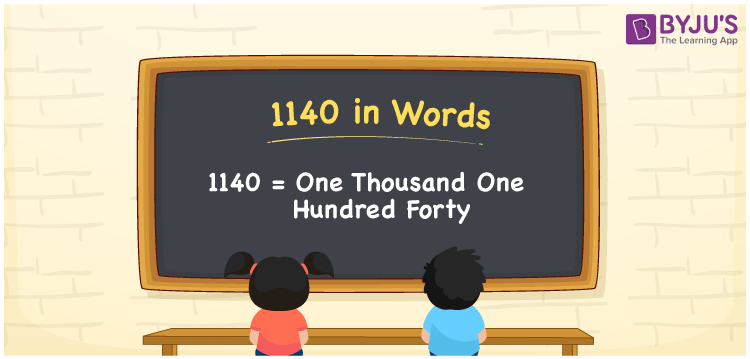# 1140 in Words

We can write 1140 in words as One thousand one hundred forty. Suppose you have written the number 1140 on a piece of paper and asked your friend to read the same, then your friend may read it as “One thousand one hundred forty”. That means the word form of the number 1140 is used to express or spell or convey the same to others. It is possible to write word forms of all cardinal numbers. In this article, you will learn to convert 1140 into words using a place value chart.

 1140 in words One thousand one hundred forty One thousand one hundred forty in numerals 1140

## 1140 in English Words

Using the English alphabet, we can express numbers in words. So, we can read 1140 in words as “One thousand one hundred forty”.## How to Write 1140 in Words?

Let us create the place value chart with four columns since the number 1140 has four digits. The below table shows the place values for all the four digits of 1140.

 Thousands Hundreds Tens Ones 1 1 4 0

Here, ones = 0, tens = 4, hundreds = 1, and thousands = 1.

These digits can be expanded as per their place values as:

1 × Thousand + 1 × Hundred + 4 × Ten + 0 × One

= 1 × 1000 + 1 × 100 + 4 × 10 + 0 × 1

= 1000 + 100 + 40

= One thousand + One hundred + Forty

= One thousand one hundred forty

Hence, 1140 in words = One thousand one hundred forty.

1140 is a natural number that precedes 1141 and succeeds 1139.

1140 in words – One thousand one hundred forty

Is 1140 an odd number? – No

Is 1140 an even number? – Yes

Is 1140 a prime number? – No

Is 1140 a composite number? – Yes

Is 1140 a perfect square number? – No

Is 1140 a perfect cube number? – No

## Frequently Asked Questions on 1140 in Words

Q1

### How do you write 1140 in words?

We can write the number 1140 in words as One thousand one hundred forty.
Q2

### How to write Rs. 1140 in words on a cheque?

On a cheque, we write Rs. 1140 in words as “One thousand one hundred forty rupees only”.
Q3

### What is the number name for 1140?

The number name for 1140 is One thousand one hundred forty.
Test your Knowledge on 1140 in Words